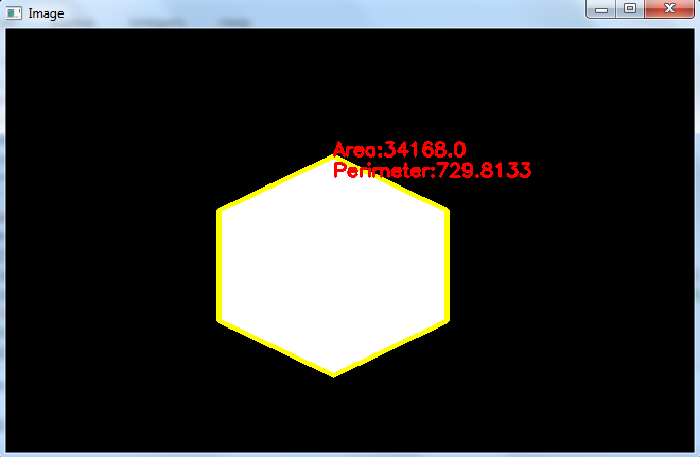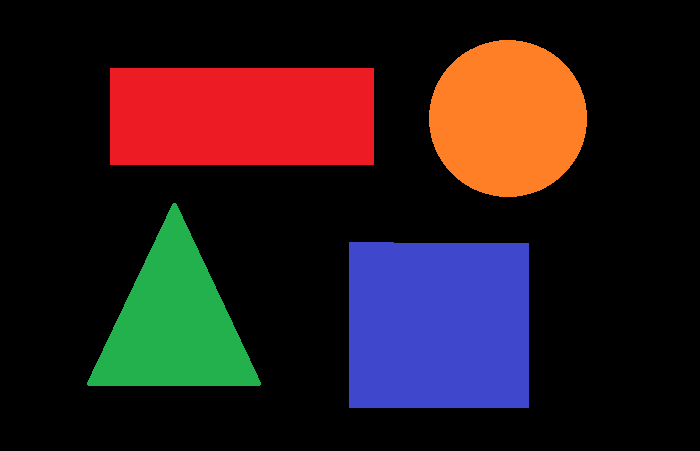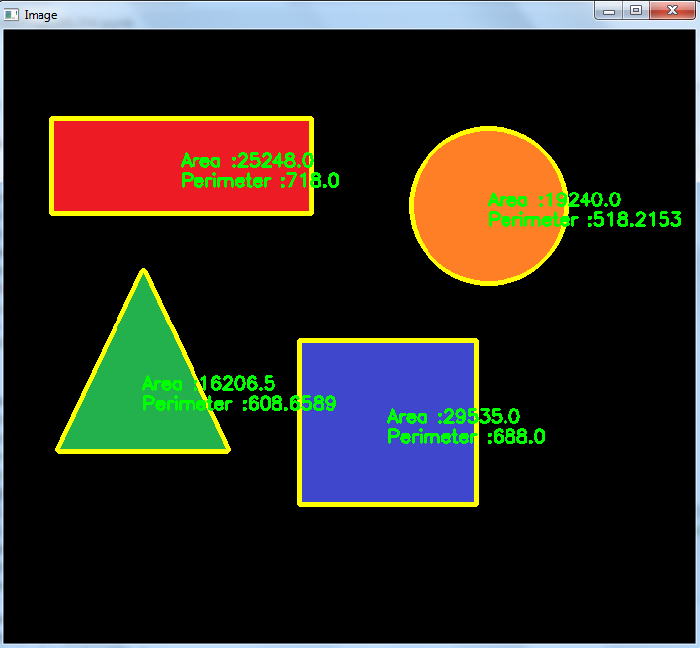# How to compute the area and perimeter of an image contour using OpenCV Python?

The contours of the objects in an image are very helpful to compute the area and perimeter of the image. A contour of an image is a curve joining all the continuous points along the boundary, having the same color or intensity. Contours are used for shape analysis and object detection and recognition etc.

To compute the area and perimeter of an object, we first detect the contour of the object and then apply cv2.contourArea() and cv2.arcLength() functions respectively.

## Syntax

The following syntax are used for the functions −

area = cv2.contourArea(cnt)
perimeter = cv2.arcLength(cnt, True)


Where, "cnt" is a numpy array of the contour points of an object in the image.

## Steps

You can use the following steps to compute the area and perimeters of the contours in an image −

Import the required library. In all the following Python examples, the required Python library is OpenCV. Make sure you have already installed it.

import cv2


img = cv2.imread('pentagon.png')
gray = cv2.cvtColor(img, cv2.COLOR_BGR2GRAY)


Apply thresholding on the grayscale image to create a binary image.

ret,thresh = cv2.threshold(gray,150,255,0)


Find the contours in the image using cv2.findContours() function.

contours, _ = cv2.findContours(thresh, cv2.RETR_TREE, cv2.CHAIN_APPROX_SIMPLE)


Compute the area and perimeters for the detected contours in the image using cv2.contourArea(cnt) and cv2.arcLength(cnt, True) functions.

area = cv2.contourArea(cnt)
perimeter = cv2.arcLength(cnt, True)


Draw the contours on the input image.

cv2.drawContours(img, [cnt], -1, (0,255,255), 3)


Print the area and perimeters of the detected contours in the image.

print('Area:', area)
print('Perimeter:', perimeter)


Let's have a look at some examples for a better understanding.

## Example 1

In this program, we compute the area and perimeter of detected contour in the input image 'pentagon.png'.

import cv2

# convert the image to grayscale
gray = cv2.cvtColor(img, cv2.COLOR_BGR2GRAY)

# Apply thresholding in the gray image to create a binary image
ret,thresh = cv2.threshold(gray,150,255,0)

# Find the contours using binary image
contours,hierarchy = cv2.findContours(thresh, cv2.RETR_TREE,cv2.CHAIN_APPROX_SIMPLE)
print("Number of contours in image:",len(contours))
cnt = contours

# compute the area and perimeter
area = cv2.contourArea(cnt)
perimeter = cv2.arcLength(cnt, True)
perimeter = round(perimeter, 4)
print('Area:', area)
print('Perimeter:', perimeter)
img1 = cv2.drawContours(img, [cnt], -1, (0,255,255), 3)
x1, y1 = cnt[0,0]
cv2.putText(img1, f'Area:{area}', (x1, y1), cv2.FONT_HERSHEY_SIMPLEX, 0.6, (0, 0, 255), 2)
cv2.putText(img1, f'Perimeter:{perimeter}', (x1, y1+20), cv2.FONT_HERSHEY_SIMPLEX, 0.6, (0, 0, 255), 2)

cv2.imshow("Image", img)
cv2.waitKey(0)
cv2.destroyAllWindows()


We will use the following image as the Input File in the above program.## Output

Running the above code gives us the following output −

Number of contours in image: 1
Area: 39738.5
Perimeter: 787.0265


And we get the following window, showing the output −## Example 2

In the below program, we compute the area and perimeter for the contours in the image. We detect four contours in the image 'shapes.jpg'.

import cv2
import numpy as np

img = cv2.cvtColor(img1, cv2.COLOR_BGR2GRAY)
ret,thresh = cv2.threshold(img,10,255,0)
contours,hierarchy = cv2.findContours(thresh, 1, 2)
print("Number of contours in image:",len(contours))

for i, cnt in enumerate(contours):
M = cv2.moments(cnt)
if M['m00'] != 0.0:
x1 = int(M['m10']/M['m00'])
y1 = int(M['m01']/M['m00'])
area = cv2.contourArea(cnt)
perimeter = cv2.arcLength(cnt, True)
perimeter = round(perimeter, 4)
print(f'Area of contour {i+1}:', area)
print(f'Perimeter of contour {i+1}:', perimeter)
img1 = cv2.drawContours(img1, [cnt], -1, (0,255,255), 3)
cv2.putText(img1, f'Area :{area}', (x1, y1), cv2.FONT_HERSHEY_SIMPLEX, 0.6, (0, 255, 0), 2)
cv2.putText(img1, f'Perimeter :{perimeter}', (x1, y1+20), cv2.FONT_HERSHEY_SIMPLEX, 0.6, (0, 255, 0), 2)

cv2.imshow("Image", img1)
cv2.waitKey(0)
cv2.destroyAllWindows()


We will use the following image as the Input File in the above program.## Output

On execution, it will produce the following output on the console −

Number of contours in image: 4
Area of contour 1: 29535.0
Perimeter of contour 1: 688.0
Area of contour 2: 16206.5
Perimeter of contour 2: 608.6589
Area of contour 3: 19240.0
Perimeter of contour 3: 518.2153
Area of contour 4: 25248.0
Perimeter of contour 4: 718.0


And we get the following window, showing the output.Updated on: 28-Sep-2022

10K+ Views